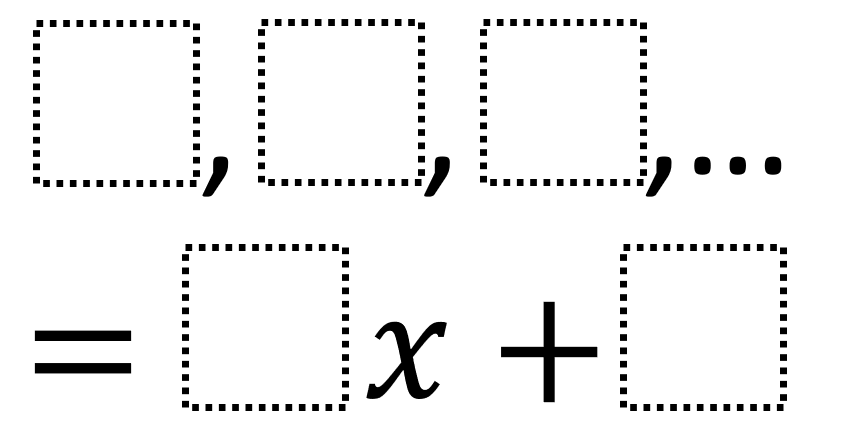# Arithmetic Sequences 2

Directions: Using the integers −9 to 9 at most one time each, place an integer in each box to create an arithmetic sequence so that the coefficient in the function that represents it is the greatest possible value.### Hint

How can you shift a sequence so that you can use the integers you have available?